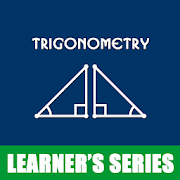# Trigonometry MathematicsEveryone
971
Application designed for beginners to learn the basics of trigonometry. This explains completely its mathematical and geometrical interpretation and physical significances. Deals all about trigonometric formulas and identities. Very helpful tutorials for students to solve mathematical problems under the category of heights and distances…try out now…

✔ Introduction Trigonometry
✔ Angle (Trigonometry)
✔ Quadrant Angle (Trigonometry)
✔ Co-terminal angles
✔ Trig functions of large and negative angles
✔ Reference angle
✔ Trigonometry functions - introduction
✔ SOH CAH TOA - trigonometry
✔ Inverse trigonometry functions
✔ Trig functions of large and negative angles
✔ Sine (sin) function - Trigonometry
✔ Arcsin
✔ Graph of the sine (sin) function - Trigonometry
✔ Sine waves - Trigonometry
✔ Cosine (cos) function - Trigonometry
✔ Arccos
✔ Graph of the cosine (cos) function - Trigonometry
✔ Tangent (tan) function - Trigonometry
✔ Arctan
✔ Graph of the tangent (tan) function - Trigonometry
✔ Cotangent
✔ Secant (sec) - Trigonometry function
✔ Cosecant (cosec) - Trigonometry function
Collapse

Review Policy
4.2
971 total
5
4
3
2
1

Updated
May 22, 2018
Size
4.0M
Installs
100,000+
Current Version
1.5.2
Requires Android
4.1 and up
Content Rating
Everyone
Permissions
Offered By
Learner's Series
Developer